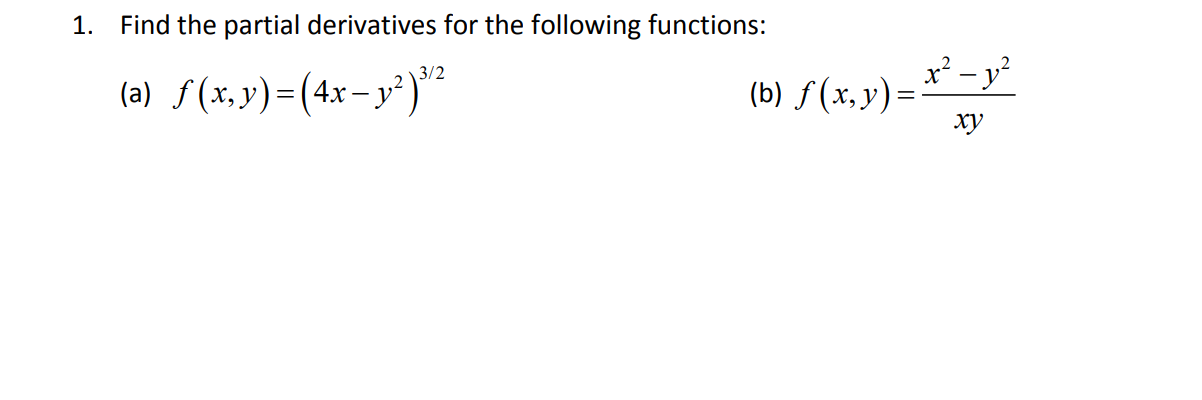# (Solved): a and b please; 1. Find the partial derivatives for the following functions: (a) f(x,y)=(4xy2)3/2 ...1. Find the partial derivatives for the following functions: (a) (b)

We have an Answer from Expert

(a)
Differentiating partially with respect to x

By using the chain rule :

Take

Using the power rule:

Substitute back

While differentiating partially with respect to x take y as constant

We have an Answer from Expert